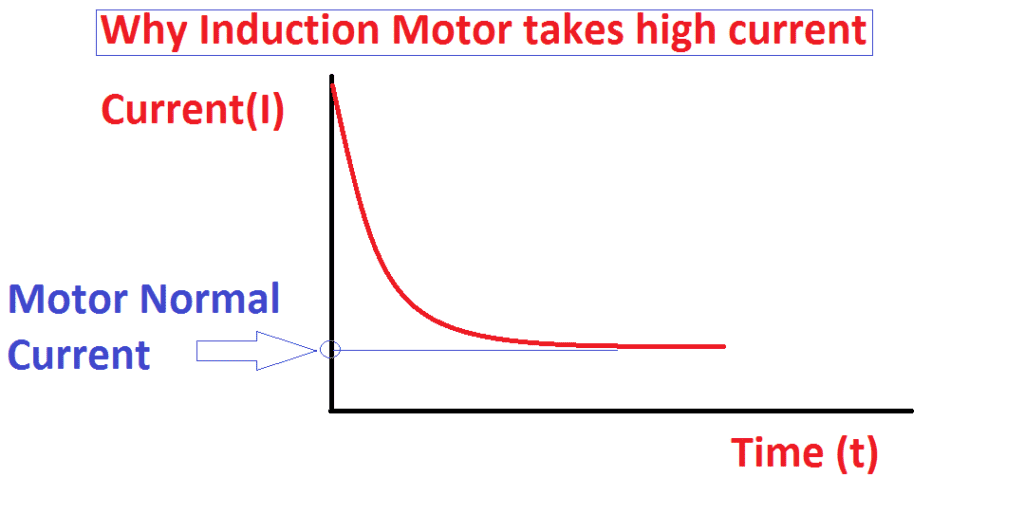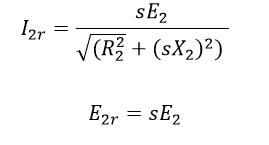# Why induction motor takes high starting current:

While starting an induction motor is the biggest headache for maintenance engineers. For this, we need to build a heavy starter or big autotransformer or VFD to start higher rating motors. In case, if the motor rating goes more than 350kw, I mean 500 HP, it will be big trouble for other sister feeders while starting the motor.

Starting of induction motor causes a heavy voltage drop in other sister feeders, rise in maximum demand at grid energy meter, the heavy magnetizing current requirement to complement this we need shunt capacitors and high current carrying capacity switchgear.

All these causes due to its starting current of the motor only, actually why starting current of the induction motor is high?…

But in case DC motor never goes higher than it full load current, but in induction motor even if you start by VFD also it will take 3 to 5 times of its full load current for few milliseconds.

Actually why… let see Why induction motor takes high starting current.

### Reason 1 Due to the inductor characteristics:

It is very simple, as we know the basic induction formula.

dV/dt=XL X dI/dt———–1

dI/dt=dV/dt X 1/XL———–2

Here…

dV/dt is changing in voltage with respect to time

XL is inductive reactance of the Motor coil

dI/dt is changing current with respect to time

Let find the current at starting, I mean what is current when t=0? To get answer for this we need to find the inductive reluctance

At t=0, then inductance XL=0 because frequency=0

XL=2pi X f

XL=0

Substitute on dI/dt equation

Note: dV/dt=constant=applied voltage. we are supplying a constant voltage

Then you will get                                          dI/dt=∞That why induction motor takes high starting current.
Here the current various as per time changes. When t=0 to certain working hours then the motor current becomes I= ∞ to motor nominal load current.

This is cannot be changed. To reduce starting current, you have to reduce applied voltage as per equation number

2. You can see the graph between the starting current to time.

### Reason2 for induction motor high starting current:

Induction Motor consists of stator and rotor. Stator acts as primary of the transformer and the rotor acts as secondary of the transformer.

In this, rotor conductor short-circuited. In a three-phase Induction motor the magnitude of the induced emf depends on the slip of the induction motor.

Here the magnitude of the rotor current is depending upon the magnitude of the rotor emf. The rotor current in the running condition will beHere you can see when the slip is high the rotor current will be very high. During the starting of the induction motor, the slip is high typically s=1 when the motor speed is zero.

At the same time, The rotor emf is equal to slip times of stator applied voltage. Hence at the start, the speed of the induction motor Is zero and the slip will be maximum.

So that the magnitude of rotor induced emf is very large at the start. But As per rotor construction, the conduction in the rotors are short-circuited. The large emf circulates very high current through rotor at the start. Now take the transformer.

In that assume, the secondary of the transformer short-circuited, with that, we are applying a full voltage at primary means, the heavy circulating current would be developed in the primary windings and secondary winding of the transformer.

As the secondary current is high, like that the primary current also is high.

Similar phenomena are considered in the three-phase induction motor also. When the rotor current is high, at the same time the stater draws high current from the input power supply.

1.Prasad
•electrical